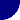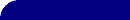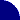# Inductance - Low Frequency LoopsRESOURCES > EIS > INDUCTANCE > LOW FREQ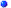Inductance and Inductive Loops
Inductive behavior which appears in the high frequency range is fairly easily explained by instrumental artifacts, or by the inductance of the electrode, or the inductance of the connecting wires. Inductive behavior at LOW frequencies, however, still mystifies many. Here are some possible explanations.A Nyquist plot, similar to that shown on the right, is occasionally seen in the impedance literature.  Although the two arcs appear to be semicircles, they are often distorted near the rightmost extreme in Z'. The point of maximum Z' often occurs above the x-axis, in the first quadrant, not on the x-axis as is shown here. The segment shown in red identifies the region of low frequency inductive behavior. An equivalent circuit that might fit this data is shown below.A Nyquist plot showing low frequency inductive behavior. The arrows point toward higher frequencies.Depending on the relative magnitudes of the time constants R2C and L/(R2+R3), the "diameter" of the red arc in the Nyquist plot, above, may be anywhere from zero to R3. The position of the "middle frequency" x-axis intercept (indicated by the "?")  be as small as R1+R2 [ when L/R3<<R2C ] or as large as (R1+R2+R3) [ when L/R3>>(R2+R3)C ].Large Inductance Values!
In contrast to the "high frequency" inductor, the value of L which fits this data is in the range of one Henry, or perhaps larger. Values this large can not be attributed to stray inductance or coiled wires. Inductances of this magnitude are characteristic of an electronic iron-core power transformer the size of a 10 cm (4 inch) cube weighing a kilogram or more!Adsorption? (MORE)
The most quoted explanation for this low frequency inductive behavior is an adsorption process at the electrode surface. I recently came across a few papers which deal with inductance, including one which details an instrumental artifact that can yield low frequency inductive behavior!More About ... Inductance at High Frequency Fitting EIS dataMore About EIS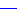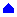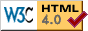© Copyright 2001-2014 Research Solutions and Resources LLC